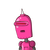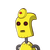# Find the perimeter of the triangle whose vertices are (-2, 1), (4, 6) and (6, -3).​

Find the perimeter of the triangle whose vertices are
(-2, 1), (4, 6) and (6, -3).​

### 2 thoughts on “Find the perimeter of the triangle whose vertices are<br /> (-2, 1), (4, 6) and (6, -3).​”

1.$$\sqrt{80} + \sqrt{61} + \sqrt{85}$$

Step-by-step explanation:

Perimeter means sum of all Sides.

So, distance between two points (a,b) and (c,d) is defined as

$$\sqrt{ {(c – a)}^{2} + \: {(d – b)}^{2} }$$

Assumptions:

Let A = (-2,1) , B = (4,6) , C = (6,-3) and AB , BC, CA be the Sides of the Triangle

Finding distance between them:

AB = $$\sqrt{ {(4 + 2)}^{2} + {(6 – 1)}^{2} }$$

= $$\sqrt{36 + 25}$$

= $$\sqrt{61}$$

BC = $$\sqrt{ {(6 – 4)}^{2} + {( – 3 – 6)}^{2} }$$

= $$\sqrt{4 + 81}$$

= $$\sqrt{85}$$

CA = $$\sqrt{ {(6 + 2)}^{2} + {( – 3 – 1)}^{2} }$$

= $$\sqrt{64 + 16}$$

= $$\sqrt{80}$$

Result:

Perimeter = Sum of all sides

= $$\sqrt{80} + \sqrt{61} + \sqrt{85}$$

2.The perimeter of a triangle is the sum of the lengths of its three sides.

To find the length of each side, we use distance formula.

Let A = (-2, 1)

Let B = (4, 6)

Let C = (6, -3)

Distance AB = $$\sqrt{(4+2)^{2} +(6-1)^{2} } = \sqrt{36+25} = \sqrt{61}$$ units

Distance BC = $$\sqrt{(6-4)^{2}+(-3+6)^{2}} = \sqrt{4+9} = \sqrt{13}$$ units

Distance CA = $$\sqrt{(6+2)^2+(-3-1)^2} = \sqrt{64+16} = \sqrt{80}$$ units

The required answer is the sum of these three values:

$$\sqrt{61} + \sqrt{13} + \sqrt{80}$$ units

Do mark as brainliest if it helped!